Subject:
Numbers and Operations
Material Type:
Lesson Plan
Level:
Middle School
7
Provider:
Pearson
Tags:
Language:
English
Media Formats:
Text/HTML

# Model Integer Subtraction## Overview

Students use the Hot Air Balloon simulation to model integer subtraction. They then move to modeling subtraction on a number line. They use patterns in their work and their answers to write a rule for subtracting integers.

# Key Concepts

This lesson introduces the number line model for subtracting integers. To subtract on a number line, start at 0. Move to the location of the first number (the minuend). Then, move in the negative direction (down or left) to subtract a positive integer or in the positive direction (up or right) to subtract a negative integer. In other words, to subtract a number, move in the opposite direction than you would if you were adding it.

The Hot Air Balloon simulation can help students see why subtracting a number is the same as adding the opposite:

• Subtracting a positive number means removing heat from air, which causes the balloon to go down, in the negative direction.
• Subtracting a negative number means removing weight, which causes the balloon to go up, in the positive direction.

The rule for integer subtraction (which extends to addition of rational numbers) is easiest to state in terms of addition: to subtract a number, add its opposite. For example, 5 – 2 = 5 + (–2) = 3 and 5 – (–2) = 5 + 2 = 7.

# Goals and Learning Objectives

• Model integer subtraction on a number line.
• Write a rule for subtracting integers.

# Teacher Demonstration

Demonstrate how to use the Hot Air Balloon interactive to model −3 − (−7) as students follow along.

• Place the balloon at 0.
• To model the minuend, –3, add 3 units of weight and move the balloon.
• To model subtracting –7, remove 7 units of weight and move the balloon.

The position of the balloon will be 4. So, −3 − (−7) = 4.

# Subtracting a Negative

Discuss the following with your classmates.

In this lesson, you will model subtraction of integers as “taking away.” In Lesson 4, you will look at subtraction in a different way—as the distance between two points.

• Use the Hot Air Balloon interactive to model subtraction as taking away:
• Start at 0 and add heat to air (for a positive number) or weight (for a negative number) to move the balloon to the first number (the minuend).
• To subtract the second number (the subtrahend) from the first number, remove or take away units of heat or weights.
• For example, to model −3 − (−7):
• Start at 0 and add 3 units of weight to move the balloon to –3. To subtract –7, remove or take away 7 units of weight.

INTERACTIVE: Hot Air Balloon

# Lesson Guide

Students should work in pairs to solve the remaining subtraction problems. Remind them to put the balloon back at 0 before each problem. Briefly review the answers and discuss the two questions about removing heat and weight. Students should have noted that removing heat (subtracting a positive) causes the balloon to go down and removing weight (subtracting a negative) causes the balloon to go up.

• −3 − (−7) = 4
• 9 − (−9) = 18
• −2 − 5 = −7
• 2 − 8 = −6
• Removing heat causes the balloon to sink.
• Removing weight causes the balloon to rise.

# Model Subtraction

Use the Hot Air Balloon interaction to model each problem and find the difference. Write your answer as an equation.

• Subtract a negative from a negative.
For example: −3 − (−7)
• Subtract a negative from a positive.
For example: 9 − (−9)
• Subtract a positive from a negative.
For example: −2 − 5
• Subtract a positive from a positive.
For example: 2 − 8

• When you remove heat from air (subtract a positive number), what happens to the balloon?

• When you remove weight (subtract a negative number), what happens to the balloon?

INTERACTIVE: Hot Air Balloon

# Lesson Guide

Removing heat (subtracting a positive number) causes the balloon to sink (move down the number line).

Removing weight (subtracting a negative number) causes the balloon to rise (move up the number line).

# On a Number Line

Discuss the following with your classmates.

You can also use a horizontal or vertical number line to model subtraction of integers as taking away.

Just as with the balloon simulation:

• Subtracting a positive number (heat) means moving in a negative direction (down or to the left).
• Subtracting a negative number (weight) means moving in a positive direction (up or to the right).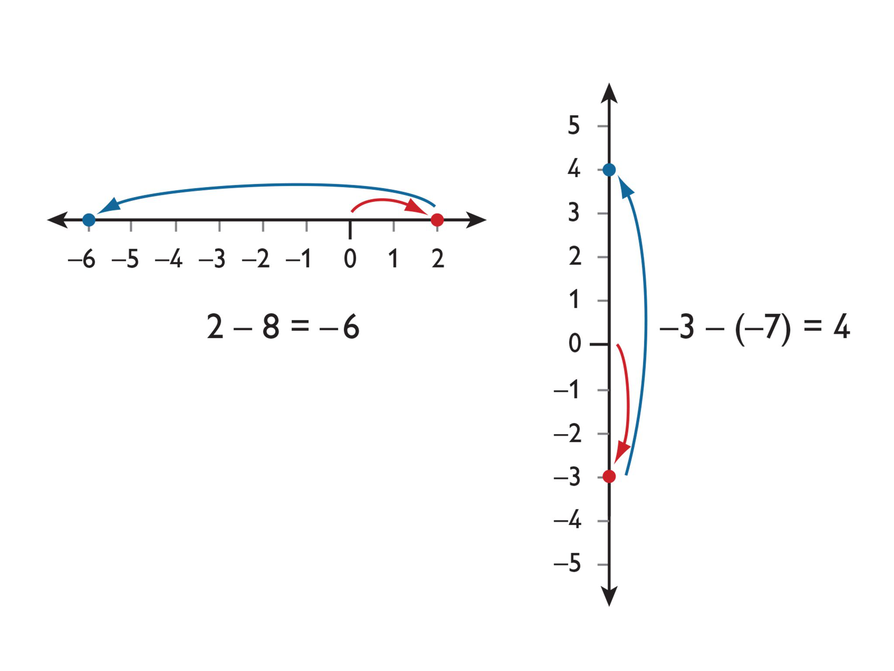# Math Mission

Discuss the Math Mission. Students will model integer subtraction on a number line and analyze guidelines for subtracting integers.

ELL: When forming partnerships, consider the task at hand. Since the work is less language dependent and more computational, pair students together that share the same language of origin, to allow for discussion.

## Opening

Model integer subtraction on a number line and analyze guidelines for subtracting integers.

# Lesson Guide

Have students work in pairs on the problems.

SWD: Struggling students may still need explicit instruction and guided scaffolding to recognize the subtraction rule. Provide small group instruction to help them subtract integers with or without the use of the Number Line tool.

# Interventions

Student has difficulty modeling subtraction problems.

• Be sure to start at 0.
• Draw an arrow to show the first number. In which direction should it point? How long should it be?
• To subtract the second number, you move in the opposite direction than you would if you were adding. Remember subtraction is the opposite of addition.
• Look at the second number. In which direction should the arrow point? How long should it be?
• Where does the second arrow end? That value is the answer.

• 7 − 4 = 3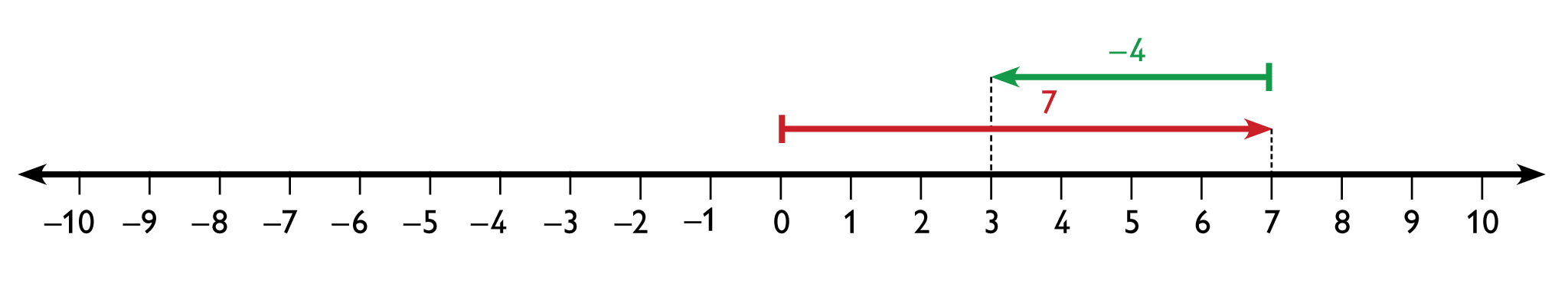• 3 − 10 = −7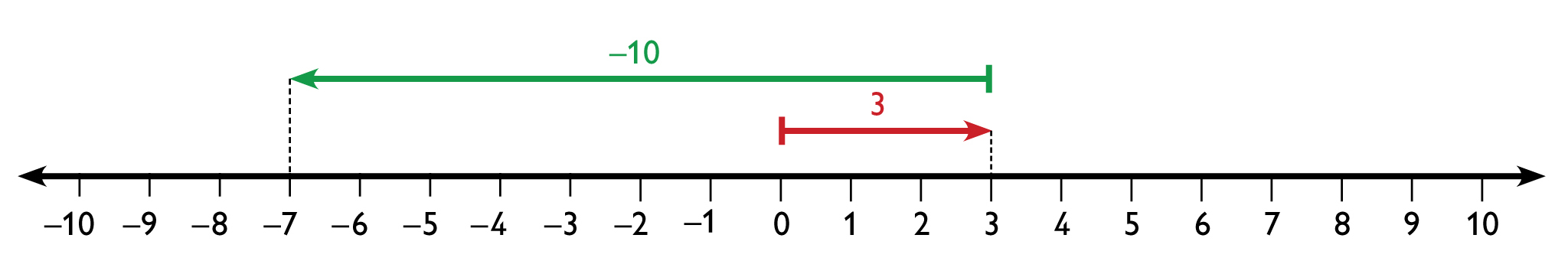• −5 − (−9) = 4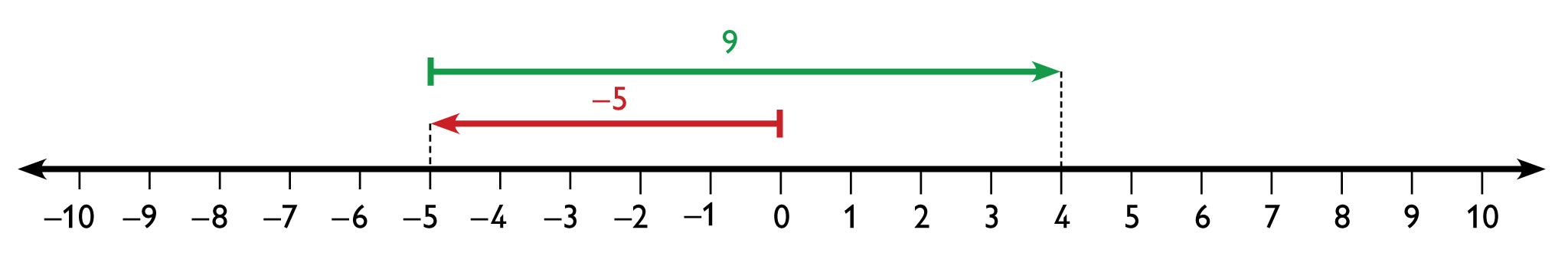• 1 − (−5) = 6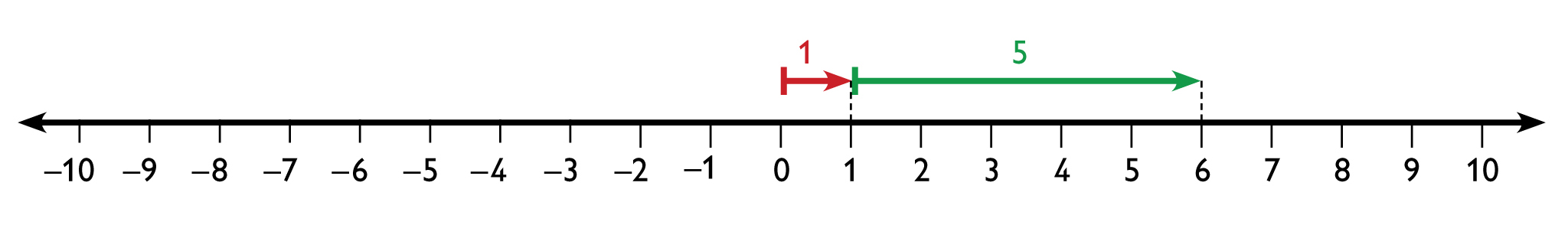• −2 − 3 = −5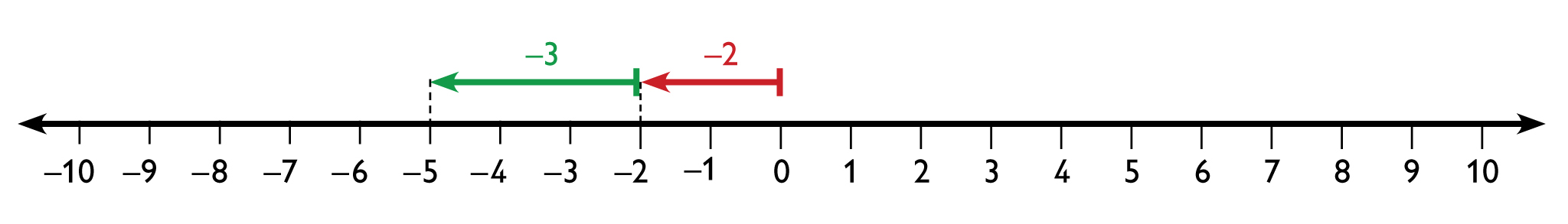• −4 − (−4) = 0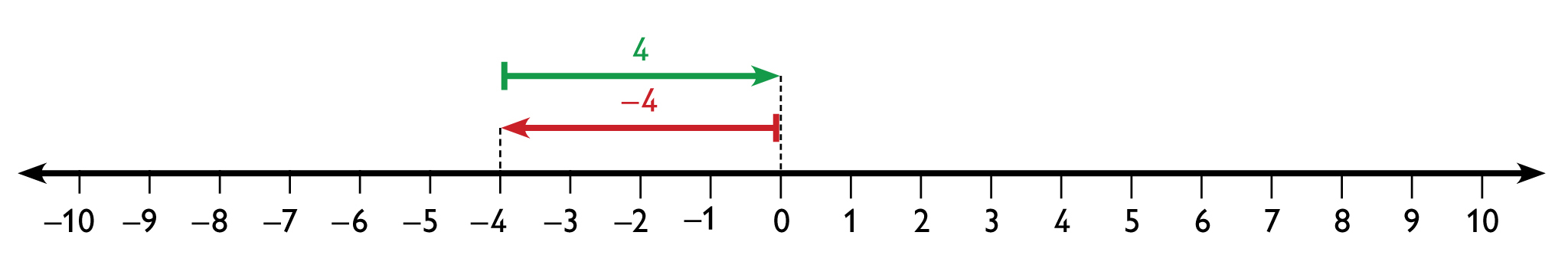• −7 − (−6) = −1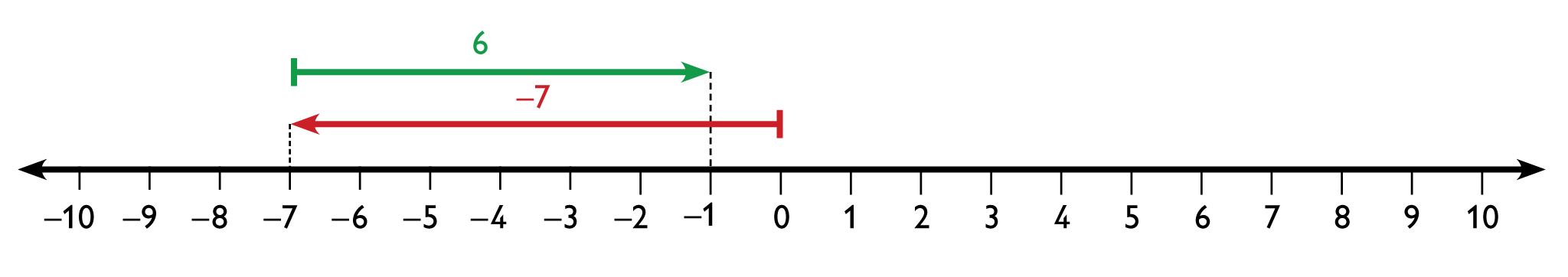# Subtract Integers on a Number Line

Model each subtraction problem on the number line. Write your answer as an equation.

• 7 − 4
• 3 − 10
• −5 − (−9)
• 1 − (−5)
• −2 − 3
• −4 − (−4)
• −7 − (−6)
• Create your own equation by subtracting any two integers.

INTERACTIVE: Number Line Tool

## Hint:

To figure out which direction to go in order to subtract the second number, think back to the balloon model. Think of subtracting a positive number as removing heat from air and subtracting a negative number as removing weight.

# Lesson Guide

Have students continue to work in pairs.

# Mathematics

When rewriting the subtraction problems as addition problems, make sure students see that each one corresponds to a problem in the first set of problems they solved with a number line. Encourage students to look at the number line for each problem to figure out how to rewrite the problems.

# Interventions

Student has difficulty writing the subtractions as additions.

• Look at the number line for the subtraction problem.
• How can you express the movements represented by the arrows as an addition problem?

• 7 − 4 = 7 + (−4)
• 3 − 10 = 3 + (−10)
• −5 − (−9) = −5 + 9
• 1 − (−5) = 1 + 5

## Work Time

Subtraction is the inverse of addition. So, subtraction problems can be written adding the opposite of the number. Subtracting a positive 2 is the same as adding a negative 2.

For example: 8 − (−2) = 8 + 2 = 10

Write these subtraction problems as addition problems:

• 7 − 4 = 7 + ____
• 3 − 10 = 3 + ____
• −5 − (−9) = −5 + ____
• 1 − (−5) = 1 + ____

## Hint:

In the first problem, when you subtract 4 on the number line, you go 4 units to the left. What addition does this represent?

# Mathematical Practices in Action

Mathematical Practice 2: Reason abstractly and quantitatively.

Have students watch the video and listen to the dialogue between Karen and Maya. This video shows students engaged in Mathematical Practice 2: Reason abstractly and quantitatively.

Look for examples of students using reasoning as they talk about the discussion that Karen and Maya had and as they try to make a generalization about subtracting integers.

# Use Reasoning

Watch the video that shows Karen and Maya use reasoning as they discuss how to subtract positive and negative numbers.

• What reasoning did Karen use to convince Maya that 6 − (−2) is not 4?
• How did the number line help Karen explain her reasoning?
• Can you make a generalization about subtracting integers?

VIDEO: Mathematical Practice 2

## Hint:

Your generalization should involve changing a subtraction problem to an addition problem.

# Preparing for Ways of Thinking

Look for students who wrote a generalization about subtraction in different ways as well as for students who wrote incorrect ones. Have these students share during Ways of Thinking. Students should be able to defend their rules and support them with examples.

ELL: For ELLs, participating in a whole class discussion such as Ways of Thinking can be intimidating for a variety of reasons. However, it is important for students to work on the speaking and listening skills implicit to this portion of the lesson. Consider these possible supports for students:

• Before students share in the whole class setting, give them a few minutes to discuss their ideas with a partner or small group. Have them discuss the questions posed and what they learned in the lesson.
• Conference with individual students prior to the discussion to ascertain what they might be able to successfully contribute to the discussion. Give students time to rehearse their contribution or to write notes for themselves to refer to when they speak. This will support students with expressive language difficulties and/or students who are anxious or reluctant to participate in class discussions.

# Challenge Problem

• n must be greater than 5.
• n must be less than 5.
• n must be greater than –5.
• n must be less than –5.

# Challenge Problem

Suppose n is an integer.

• If 5 − n is negative, what do you know about the value of n?
• If 5 − n is positive, what do you know about the value of n?
• If −5 − n is negative, what do you know about the value of n?
• If −5 − n is positive, what do you know about the value of n?

# Lesson Guide

Work as a class to correct any incorrect generalizations about subtracting integers. At the end of the discussion, the class should agree on a version of the subtraction rule that everyone understands and can apply.

Include a conversation of the Work Time video in the discussion. The video shows students involved in a discussion that illustrates Mathematical Practice 2: Reason abstractly and quantitatively. Ask students to evaluate Karen’s reasoning and have them discuss if her reasoning helped them create a generalization about subtracting integers.

# Ways of Thinking: Make Connections

Take notes about your classmates’ approaches to subtracting positive and negative numbers.

## Hint:

• How did you know which direction to go for the second number?
• Can you show me an example to help me understand your generalization?
• Did you test your generalization to make sure it works for all the subtraction problems that you wrote as addition problems earlier in the lesson?
• Why does subtracting a negative number always result in an answer that is greater than the first number?

# Lesson Guide

Have students work individually on the problems. Help students who have difficulty applying the subtraction rule.

• 123 − 4 = 119
• 3 − 123 = −120
• −5 − (−23) = 18
• 23 − (−15) = 38

# Apply the Learning: Practice Subtracting Integers

Solve the problems. Write each solution as an equation.

• 123 − 4
• 3 − 123
• −5 − (−23)
• 23 − (−15)

# Lesson Guide

Have students discuss the summary before leading a whole class discussion. Compare the class subtraction rule with the one presented.

ELL: Asking students to reflect on their learning provides opportunities for ELLs to develop literacy in English and proficiency in mathematics. Make sure students use both academic and specialized mathematical language when reflecting on their learning at the end of each session.

# Summary of the Math: Subtract Integers

The value of ab is:

• Positive if a > b (if a is to the right of b on the number line).

• Negative if a < b (if a is to the left of b on the number line).

Any subtraction problem can be rewritten as an addition problem by adding the opposite: ab = a + (–b)

## Hint:

• Explain what it means to subtract integers by taking away?
• Describe how to subtract integers on a number line?
• Explain how to subtract any two integers?
• Explain the guideline for using addition to subtract any two integers?